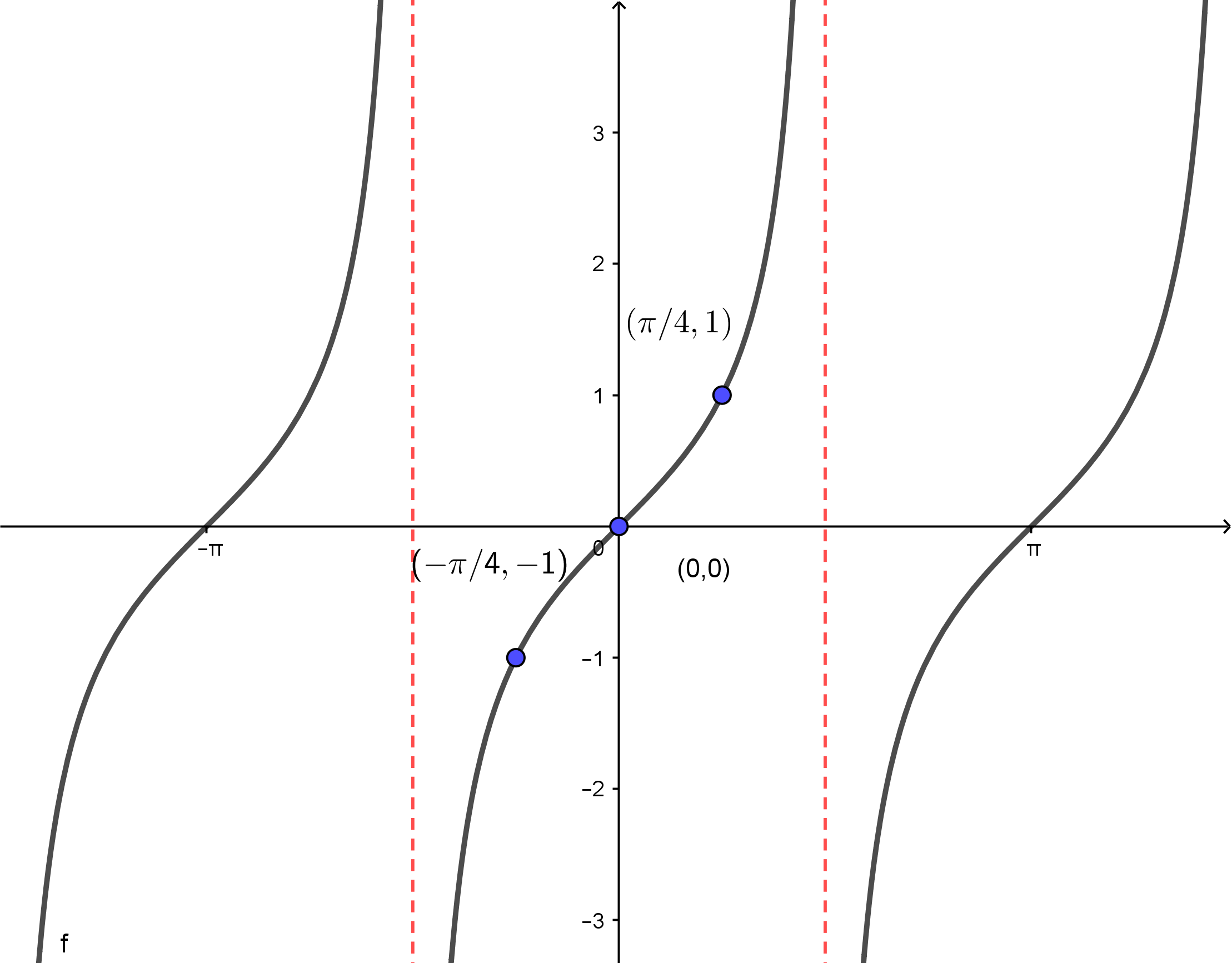# Section 1.6 Graphs of the Other Trig Functions

Section Objectives

1. Sketch the graphs of general tangent functions.
2. Sketch the graphs of general secant, cosecant, and cotangent functions.

### Graph of the Tangent Function

When thinking about the tangent function, here are the main points to keep in mind:

• Domain: $y=\tan x$ is not defined at any odd multiple of $\pi/2$. (Think about the unit circle!)
• Range: The output values range from $-\infty$ to $+\infty$.
• Periodic: The tangent has period $\pi$. The graph repeats every $\pi$ units.
• Symmetry: The tangent exhibits odd symmetry: $\tan(-x) = -\tan(x)$. The graph is symmetric about the origin.
• Asymptotes: The graph has a vertical asymptote at every odd multiple of $\pi/2$.
• Points: A number of specific points on the graph of each function can be obtained from the unit circle.

The graph of $y=\tan x$ is shown here.### More General Graphs of Tangent

For graphing general tangent functions, we can adopt strategies similar to those used for graphing sines and cosines. The general form of the tangent function is

$y = d + a \tan(bx-c)$

• To get the general shape, place vertical asymptotes at the $x$-values that satisfy

$\displaystyle bx-c = -\frac{\pi}{2}$ and $\displaystyle bx-c=\frac{\pi}{2}$.

Other vertical asymptotes then repeat with the same spacing.

• The "middle" of a period is the midpoint of your $x$-values above.

• $d$ represents a vertical shift.

• $a$ is a scale factor (similar to amplitude). If $a<0$, the graph is flipped about the $x$-axis.

#### Examples

• Sketch the graph of $y=2 \tan x$

• Sketch the graph of $\displaystyle y=1+2\tan \frac{x}{2}$

• Sketch the graph of $y=1-\cos x$

### Graphs of the Other Remaining Trig Functions

To graph any other trig function, we will first graph the corresponding reciprocal function, place vertical asymptotes at all zeros, and think about reciprocal values. It's actually pretty easy to do this once we've master sine, cosine, and tangent.

Rather than explain in writing, we will do lots of examples.

#### Examples

• Sketch the graph of $\displaystyle y=2 \cot \frac{x}{3}$.

• Sketch the graph of $\displaystyle y=2 \csc\left(x-\frac{\pi}{4} \right)$.

• Sketch the graph of $\displaystyle y=2-2 \sec 4x$.